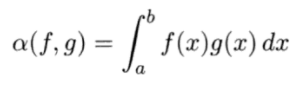# Bilinear Function

A bilinear function (or bilinear form) is a function that’s bilinear for all arguments, which can be scalar or vector (Vinberg, 2003; Haddon, 2000). In other words, it is a linear function of x for every fixed y-value and a linear function of y for every x-value (Shilov & Silverman, 1963).

## Bilinear Function Formula and Examples

The functionis bilinear for every argument on the space ℂ[a, b], where:

A bilinear form can also be defined in terms of matrices. Every bilinear function on ℝ (the reals) has the form
(x, y) = xtAy
for some n x n matrix A.

A couple of specific bilinear function examples (Karageorgis, 2020):

• An inner product on real-numbered vector space V; This bilinear form is positive definite and symmetric (its variables are unchanged under any permutation; In other words, if you switch out two of the variables, you end up with the same function),
• The dot product on ℝ is a bilinear function.

## Related Functions

A symmetric bilinear function is where f(u, v) = f(v, u) for all u and v. Multilinear functions are a generalization of bilinear functions; generally speaking, differential forms are alternating multilinear functions (Harvard, 2017).

## References

Haddon, J. 2000. Shape Representation and Bilinear Problems in Computer Vision. University of California, Berkeley.